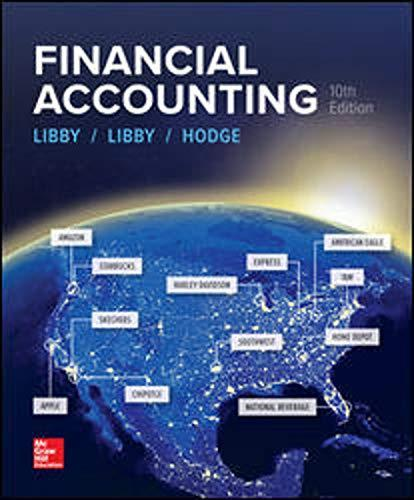# Straight-Line Depreciation A computer was purchased on January 1 at a cost of \$3,000. It has an estimated useful life of five years and an estimated salvage value of \$300. Prepare a depreciation schedule showing the depreciation expense, accumulated depreciation, and book value for each year under the straight-line method.

Question
100%

Straight-Line Depreciation

A computer was purchased on January 1 at a cost of \$3,000. It has an estimated useful life of five years and an estimated salvage value of \$300.

Prepare a depreciation schedule showing the depreciation expense, accumulated depreciation, and book value for each year under the straight-line method.

Expert Solution

### Want to see the full answer?

Check out a sample Q&A hereStudents who’ve seen this question also like:FINANCIAL ACCOUNTING
10th Edition
ISBN: 9781259964947
Author: Libby
Publisher: MCG